Intermediate Algebra

# 7.2Add and Subtract Rational Expressions

Intermediate Algebra7.2 Add and Subtract Rational Expressions

### Learning Objectives

By the end of this section, you will be able to:
• Add and subtract rational expressions with a common denominator
• Add and subtract rational expressions whose denominators are opposites
• Find the least common denominator of rational expressions
• Add and subtract rational expressions with unlike denominators
• Add and subtract rational functions

### Be Prepared 7.2

Before you get started, take this readiness quiz.

1. Add: $710+815.710+815.$
If you missed this problem, review Example 1.29.
2. Subtract: $3x4−89.3x4−89.$
If you missed this problem, review Example 1.28.
3. Subtract: $6(2x+1)−4(x−5).6(2x+1)−4(x−5).$
If you missed this problem, review Example 1.56.

### Add and Subtract Rational Expressions with a Common Denominator

What is the first step you take when you add numerical fractions? You check if they have a common denominator. If they do, you add the numerators and place the sum over the common denominator. If they do not have a common denominator, you find one before you add.

It is the same with rational expressions. To add rational expressions, they must have a common denominator. When the denominators are the same, you add the numerators and place the sum over the common denominator.

### Rational Expression Addition and Subtraction

If p, q, and r are polynomials where $r≠0,r≠0,$ then

$pr+qr=p+qrandpr−qr=p−qrpr+qr=p+qrandpr−qr=p−qr$

To add or subtract rational expressions with a common denominator, add or subtract the numerators and place the result over the common denominator.

We always simplify rational expressions. Be sure to factor, if possible, after you subtract the numerators so you can identify any common factors.

Remember, too, we do not allow values that would make the denominator zero. What value of x should be excluded in the next example?

### Example 7.13

Add: $11x+28x+4+x2x+4.11x+28x+4+x2x+4.$

### Try It 7.25

Simplify: $9x+14x+7+x2x+7.9x+14x+7+x2x+7.$

### Try It 7.26

Simplify: $x2+8xx+5+15x+5.x2+8xx+5+15x+5.$

To subtract rational expressions, they must also have a common denominator. When the denominators are the same, you subtract the numerators and place the difference over the common denominator. Be careful of the signs when you subtract a binomial or trinomial.

### Example 7.14

Subtract: $5x2−7x+3x2−3x+18−4x2+x−9x2−3x+18.5x2−7x+3x2−3x+18−4x2+x−9x2−3x+18.$

### Try It 7.27

Subtract: $4x2−11x+8x2−3x+2−3x2+x−3x2−3x+2.4x2−11x+8x2−3x+2−3x2+x−3x2−3x+2.$

### Try It 7.28

Subtract: $6x2−x+20x2−81−5x2+11x−7x2−81.6x2−x+20x2−81−5x2+11x−7x2−81.$

### Add and Subtract Rational Expressions Whose Denominators are Opposites

When the denominators of two rational expressions are opposites, it is easy to get a common denominator. We just have to multiply one of the fractions by $−1−1.−1−1.$

Let’s see how this works.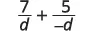Multiply the second fraction by $−1−1.−1−1.$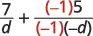The denominators are the same.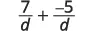Simplify.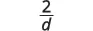Be careful with the signs as you work with the opposites when the fractions are being subtracted.

### Example 7.15

Subtract: $m2−6mm2−1−3m+21−m2.m2−6mm2−1−3m+21−m2.$

### Try It 7.29

Subtract: $y2−5yy2−4−6y−64−y2.y2−5yy2−4−6y−64−y2.$

### Try It 7.30

Subtract: $2n2+8n−1n2−1−n2−7n−11−n2.2n2+8n−1n2−1−n2−7n−11−n2.$

### Find the Least Common Denominator of Rational Expressions

When we add or subtract rational expressions with unlike denominators, we will need to get common denominators. If we review the procedure we used with numerical fractions, we will know what to do with rational expressions.

Let’s look at this example: $712+518.712+518.$ Since the denominators are not the same, the first step was to find the least common denominator (LCD).

To find the LCD of the fractions, we factored 12 and 18 into primes, lining up any common primes in columns. Then we “brought down” one prime from each column. Finally, we multiplied the factors to find the LCD.

When we add numerical fractions, once we found the LCD, we rewrote each fraction as an equivalent fraction with the LCD by multiplying the numerator and denominator by the same number. We are now ready to add.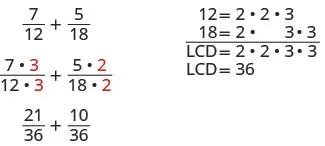We do the same thing for rational expressions. However, we leave the LCD in factored form.

### How To

#### Find the least common denominator of rational expressions.

1. Step 1. Factor each denominator completely.
2. Step 2. List the factors of each denominator. Match factors vertically when possible.
3. Step 3. Bring down the columns by including all factors, but do not include common factors twice.
4. Step 4. Write the LCD as the product of the factors.

Remember, we always exclude values that would make the denominator zero. What values of $xx$ should we exclude in this next example?

### Example 7.16

Find the LCD for the expressions $8x2−2x−3,3xx2+4x+38x2−2x−3,3xx2+4x+3$ and rewrite them as equivalent rational expressions with the lowest common denominator.

### Try It 7.31

Find the LCD for the expressions $2x2−x−12,1x2−162x2−x−12,1x2−16$ rewrite them as equivalent rational expressions with the lowest common denominator.

### Try It 7.32

Find the LCD for the expressions $3xx2−3x–10,5x2+3x+23xx2−3x–10,5x2+3x+2$ rewrite them as equivalent rational expressions with the lowest common denominator.

### Add and Subtract Rational Expressions with Unlike Denominators

Now we have all the steps we need to add or subtract rational expressions with unlike denominators.

### Example 7.17

#### How to Add Rational Expressions with Unlike Denominators

Add: $3x−3+2x−2.3x−3+2x−2.$

### Try It 7.33

Add: $2x−2+5x+3.2x−2+5x+3.$

### Try It 7.34

Add:$4m+3+3m+4.4m+3+3m+4.$

The steps used to add rational expressions are summarized here.

### How To

#### Add or subtract rational expressions.

1. Step 1.
Determine if the expressions have a common denominator.
• Yes – go to step 2.
• No – Rewrite each rational expression with the LCD.
• Find the LCD.
• Rewrite each rational expression as an equivalent rational expression with the LCD.
2. Step 2. Add or subtract the rational expressions.
3. Step 3. Simplify, if possible.

Avoid the temptation to simplify too soon. In the example above, we must leave the first rational expression as $3x−6(x−3)(x−2)3x−6(x−3)(x−2)$ to be able to add it to $2x−6(x−2)(x−3).2x−6(x−2)(x−3).$ Simplify only after you have combined the numerators.

### Example 7.18

Add: $8x2−2x−3+3xx2+4x+3.8x2−2x−3+3xx2+4x+3.$

### Try It 7.35

Add: $1m2−m−2+5mm2+3m+2.1m2−m−2+5mm2+3m+2.$

### Try It 7.36

Add:$2nn2−3n−10+6n2+5n+6.2nn2−3n−10+6n2+5n+6.$

The process we use to subtract rational expressions with different denominators is the same as for addition. We just have to be very careful of the signs when subtracting the numerators.

### Example 7.19

Subtract: $8yy2−16−4y−4.8yy2−16−4y−4.$

### Try It 7.37

Subtract: $2xx2−4−1x+2.2xx2−4−1x+2.$

### Try It 7.38

Subtract: $3z+3−6zz2−9.3z+3−6zz2−9.$

There are lots of negative signs in the next example. Be extra careful.

### Example 7.20

Subtract:$−3n−9n2+n−6−n+32−n.−3n−9n2+n−6−n+32−n.$

### Try It 7.39

Subtract :$3x−1x2−5x−6−26−x.3x−1x2−5x−6−26−x.$

### Try It 7.40

Subtract: $−2y−2y2+2y−8−y−12−y.−2y−2y2+2y−8−y−12−y.$

Things can get very messy when both fractions must be multiplied by a binomial to get the common denominator.

### Example 7.21

Subtract: $4a2+6a+5−3a2+7a+10.4a2+6a+5−3a2+7a+10.$

### Try It 7.41

Subtract: $3b2−4b−5−2b2−6b+5.3b2−4b−5−2b2−6b+5.$

### Try It 7.42

Subtract: $4x2−4−3x2−x−2.4x2−4−3x2−x−2.$

We follow the same steps as before to find the LCD when we have more than two rational expressions. In the next example, we will start by factoring all three denominators to find their LCD.

### Example 7.22

Simplify: $2uu−1+1u−2u−1u2−u.2uu−1+1u−2u−1u2−u.$

### Try It 7.43

Simplify: $vv+1+3v−1−6v2−1.vv+1+3v−1−6v2−1.$

### Try It 7.44

Simplify: $3ww+2+2w+7−17w+4w2+9w+14.3ww+2+2w+7−17w+4w2+9w+14.$

### Add and subtract rational functions

To add or subtract rational functions, we use the same techniques we used to add or subtract polynomial functions.

### Example 7.23

Find $R(x)=f(x)−g(x)R(x)=f(x)−g(x)$ where $f(x)=x+5x−2f(x)=x+5x−2$ and $g(x)=5x+18x2−4.g(x)=5x+18x2−4.$

### Try It 7.45

Find $R(x)=f(x)−g(x)R(x)=f(x)−g(x)$ where $f(x)=x+1x+3f(x)=x+1x+3$ and $g(x)=x+17x2−x−12.g(x)=x+17x2−x−12.$

### Try It 7.46

Find $R(x)=f(x)+g(x)R(x)=f(x)+g(x)$ where $f(x)=x−4x+3f(x)=x−4x+3$ and $g(x)=4x+6x2−9.g(x)=4x+6x2−9.$

### Media

Access this online resource for additional instruction and practice with adding and subtracting rational expressions.

### Section 7.2 Exercises

#### Practice Makes Perfect

Add and Subtract Rational Expressions with a Common Denominator

75.

$2 15 + 7 15 2 15 + 7 15$

76.

$7 24 + 11 24 7 24 + 11 24$

77.

$3 c 4 c − 5 + 5 4 c − 5 3 c 4 c − 5 + 5 4 c − 5$

78.

$7 m 2 m + n + 4 2 m + n 7 m 2 m + n + 4 2 m + n$

79.

$2 r 2 2 r − 1 + 15 r − 8 2 r − 1 2 r 2 2 r − 1 + 15 r − 8 2 r − 1$

80.

$3 s 2 3 s − 2 + 13 s − 10 3 s − 2 3 s 2 3 s − 2 + 13 s − 10 3 s − 2$

81.

$2 w 2 w 2 − 16 + 8 w w 2 − 16 2 w 2 w 2 − 16 + 8 w w 2 − 16$

82.

$7 x 2 x 2 − 9 + 21 x x 2 − 9 7 x 2 x 2 − 9 + 21 x x 2 − 9$

In the following exercises, subtract.

83.

$9 a 2 3 a − 7 − 49 3 a − 7 9 a 2 3 a − 7 − 49 3 a − 7$

84.

$25 b 2 5 b − 6 − 36 5 b − 6 25 b 2 5 b − 6 − 36 5 b − 6$

85.

$3 m 2 6 m − 30 − 21 m − 30 6 m − 30 3 m 2 6 m − 30 − 21 m − 30 6 m − 30$

86.

$2 n 2 4 n − 32 − 18 n − 16 4 n − 32 2 n 2 4 n − 32 − 18 n − 16 4 n − 32$

87.

$6 p 2 + 3 p + 4 p 2 + 4 p − 5 − 5 p 2 + p + 7 p 2 + 4 p − 5 6 p 2 + 3 p + 4 p 2 + 4 p − 5 − 5 p 2 + p + 7 p 2 + 4 p − 5$

88.

$5 q 2 + 3 q − 9 q 2 + 6 q + 8 − 4 q 2 + 9 q + 7 q 2 + 6 q + 8 5 q 2 + 3 q − 9 q 2 + 6 q + 8 − 4 q 2 + 9 q + 7 q 2 + 6 q + 8$

89.

$5 r 2 + 7 r − 33 r 2 − 49 − 4 r 2 + 5 r + 30 r 2 − 49 5 r 2 + 7 r − 33 r 2 − 49 − 4 r 2 + 5 r + 30 r 2 − 49$

90.

$7 t 2 − t − 4 t 2 − 25 − 6 t 2 + 12 t − 44 t 2 − 25 7 t 2 − t − 4 t 2 − 25 − 6 t 2 + 12 t − 44 t 2 − 25$

Add and Subtract Rational Expressions whose Denominators are Opposites

In the following exercises, add or subtract.

91.

$10 v 2 v − 1 + 2 v + 4 1 − 2 v 10 v 2 v − 1 + 2 v + 4 1 − 2 v$

92.

$20 w 5 w − 2 + 5 w + 6 2 − 5 w 20 w 5 w − 2 + 5 w + 6 2 − 5 w$

93.

$10 x 2 + 16 x − 7 8 x − 3 + 2 x 2 + 3 x − 1 3 − 8 x 10 x 2 + 16 x − 7 8 x − 3 + 2 x 2 + 3 x − 1 3 − 8 x$

94.

$6 y 2 + 2 y − 11 3 y − 7 + 3 y 2 − 3 y + 17 7 − 3 y 6 y 2 + 2 y − 11 3 y − 7 + 3 y 2 − 3 y + 17 7 − 3 y$

95.

$z 2 + 6 z z 2 − 25 − 3 z + 20 25 − z 2 z 2 + 6 z z 2 − 25 − 3 z + 20 25 − z 2$

96.

$a 2 + 3 a a 2 − 9 − 3 a − 27 9 − a 2 a 2 + 3 a a 2 − 9 − 3 a − 27 9 − a 2$

97.

$2 b 2 + 30 b − 13 b 2 − 49 − 2 b 2 − 5 b − 8 49 − b 2 2 b 2 + 30 b − 13 b 2 − 49 − 2 b 2 − 5 b − 8 49 − b 2$

98.

$c 2 + 5 c − 10 c 2 − 16 − c 2 − 8 c − 10 16 − c 2 c 2 + 5 c − 10 c 2 − 16 − c 2 − 8 c − 10 16 − c 2$

Find the Least Common Denominator of Rational Expressions

In the following exercises, find the LCD for the given rational expressions rewrite them as equivalent rational expressions with the lowest common denominator.

99.

$5 x 2 − 2 x − 8 , 2 x x 2 − x − 12 5 x 2 − 2 x − 8 , 2 x x 2 − x − 12$

100.

$8 y 2 + 12 y + 35 , 3 y y 2 + y − 42 8 y 2 + 12 y + 35 , 3 y y 2 + y − 42$

101.

$9 z 2 + 2 z − 8 , 4 z z 2 − 4 9 z 2 + 2 z − 8 , 4 z z 2 − 4$

102.

$6 a 2 + 14 a + 45 , 5 a a 2 − 81 6 a 2 + 14 a + 45 , 5 a a 2 − 81$

103.

$4 b 2 + 6 b + 9 , 2 b b 2 − 2 b − 15 4 b 2 + 6 b + 9 , 2 b b 2 − 2 b − 15$

104.

$5 c 2 − 4 c + 4 , 3 c c 2 − 7 c + 10 5 c 2 − 4 c + 4 , 3 c c 2 − 7 c + 10$

105.

$2 3 d 2 + 14 d − 5 , 5 d 3 d 2 − 19 d + 6 2 3 d 2 + 14 d − 5 , 5 d 3 d 2 − 19 d + 6$

106.

$3 5 m 2 − 3 m − 2 , 6 m 5 m 2 + 17 m + 6 3 5 m 2 − 3 m − 2 , 6 m 5 m 2 + 17 m + 6$

Add and Subtract Rational Expressions with Unlike Denominators

In the following exercises, perform the indicated operations.

107.

$7 10 x 2 y + 4 15 x y 2 7 10 x 2 y + 4 15 x y 2$

108.

$1 12 a 3 b 2 + 5 9 a 2 b 3 1 12 a 3 b 2 + 5 9 a 2 b 3$

109.

$3 r + 4 + 2 r − 5 3 r + 4 + 2 r − 5$

110.

$4 s − 7 + 5 s + 3 4 s − 7 + 5 s + 3$

111.

$5 3 w − 2 + 2 w + 1 5 3 w − 2 + 2 w + 1$

112.

$4 2 x + 5 + 2 x − 1 4 2 x + 5 + 2 x − 1$

113.

$2 y y + 3 + 3 y − 1 2 y y + 3 + 3 y − 1$

114.

$3 z z − 2 + 1 z + 5 3 z z − 2 + 1 z + 5$

115.

$5 b a 2 b − 2 a 2 + 2 b b 2 − 4 5 b a 2 b − 2 a 2 + 2 b b 2 − 4$

116.

$4 c d + 3 c + 1 d 2 − 9 4 c d + 3 c + 1 d 2 − 9$

117.

$−3 m 3 m − 3 + 5 m m 2 + 3 m − 4 −3 m 3 m − 3 + 5 m m 2 + 3 m − 4$

118.

$8 4 n + 4 + 6 n 2 − n − 2 8 4 n + 4 + 6 n 2 − n − 2$

119.

$3 r r 2 + 7 r + 6 + 9 r 2 + 4 r + 3 3 r r 2 + 7 r + 6 + 9 r 2 + 4 r + 3$

120.

$2 s s 2 + 2 s − 8 + 4 s 2 + 3 s − 10 2 s s 2 + 2 s − 8 + 4 s 2 + 3 s − 10$

121.

$t t − 6 − t − 2 t + 6 t t − 6 − t − 2 t + 6$

122.

$x − 3 x + 6 − x x + 3 x − 3 x + 6 − x x + 3$

123.

$5 a a + 3 − a + 2 a + 6 5 a a + 3 − a + 2 a + 6$

124.

$3 b b − 2 − b − 6 b − 8 3 b b − 2 − b − 6 b − 8$

125.

$6 m + 6 − 12 m m 2 − 36 6 m + 6 − 12 m m 2 − 36$

126.

$4 n + 4 − 8 n n 2 − 16 4 n + 4 − 8 n n 2 − 16$

127.

$−9 p − 17 p 2 − 4 p − 21 − p + 1 7 − p −9 p − 17 p 2 − 4 p − 21 − p + 1 7 − p$

128.

$− 13 q - 8 q 2 + 2 q − 24 − q + 2 4 − q − 13 q - 8 q 2 + 2 q − 24 − q + 2 4 − q$

129.

$−2 r − 16 r 2 + 6 r − 16 − 5 2 − r −2 r − 16 r 2 + 6 r − 16 − 5 2 − r$

130.

$2 t − 30 t 2 + 6 t − 27 − 2 3 − t 2 t − 30 t 2 + 6 t − 27 − 2 3 − t$

131.

$2 x + 7 10 x − 1 + 3 2 x + 7 10 x − 1 + 3$

132.

$8 y − 4 5 y + 2 − 6 8 y − 4 5 y + 2 − 6$

133.

$3 x 2 − 3 x − 4 − 2 x 2 − 5 x + 4 3 x 2 − 3 x − 4 − 2 x 2 − 5 x + 4$

134.

$4 x 2 − 6 x + 5 − 3 x 2 − 7 x + 10 4 x 2 − 6 x + 5 − 3 x 2 − 7 x + 10$

135.

$5 x 2 + 8 x − 9 − 4 x 2 + 10 x + 9 5 x 2 + 8 x − 9 − 4 x 2 + 10 x + 9$

136.

$3 2 x 2 + 5 x + 2 − 1 2 x 2 + 3 x + 1 3 2 x 2 + 5 x + 2 − 1 2 x 2 + 3 x + 1$

137.

$5 a a − 2 + 9 a − 2 a + 18 a 2 − 2 a 5 a a − 2 + 9 a − 2 a + 18 a 2 − 2 a$

138.

$2 b b − 5 + 3 2 b − 2 b − 15 2 b 2 − 10 b 2 b b − 5 + 3 2 b − 2 b − 15 2 b 2 − 10 b$

139.

$c c + 5 + 5 c − 2 − 10 c c 2 − 4 c c + 5 + 5 c − 2 − 10 c c 2 − 4$

140.

$6 d d − 5 + 1 d + 4 − 7 d − 5 d 2 − d − 20 6 d d − 5 + 1 d + 4 − 7 d − 5 d 2 − d − 20$

141.

$3 d d + 2 + 4 d − d + 8 d 2 + 2 d 3 d d + 2 + 4 d − d + 8 d 2 + 2 d$

142.

$2 q q + 5 + 3 q − 3 − 13 q + 15 q 2 + 2 q − 15 2 q q + 5 + 3 q − 3 − 13 q + 15 q 2 + 2 q − 15$

In the following exercises, find $R(x)=f(x)+g(x)R(x)=f(x)+g(x)$ $R(x)=f(x)−g(x).R(x)=f(x)−g(x).$

143.

$f(x)=−5x−5x2+x−6f(x)=−5x−5x2+x−6$ and
$g(x)=x+12−xg(x)=x+12−x$

144.

$f(x)=−4x−24x2+x−30f(x)=−4x−24x2+x−30$ and
$g(x)=x+75−xg(x)=x+75−x$

145.

$f(x)=6xx2−64f(x)=6xx2−64$ and
$g(x)=3x−8g(x)=3x−8$

146.

$f(x)=5x+7f(x)=5x+7$ and
$g(x)=10xx2−49g(x)=10xx2−49$

#### Writing Exercises

147.

Donald thinks that $3x+4x3x+4x$ is $72x.72x.$ Is Donald correct? Explain.

148.

Explain how you find the Least Common Denominator of $x2+5x+4x2+5x+4$ and $x2−16.x2−16.$

149.

Felipe thinks $1x+1y1x+1y$ is $2x+y.2x+y.$ Choose numerical values for x and y and evaluate $1x+1y.1x+1y.$ Evaluate $2x+y2x+y$ for the same values of x and y you used in part . Explain why Felipe is wrong. Find the correct expression for $1x+1y.1x+1y.$

150.

Simplify the expression $4n2+6n+9−1n2−94n2+6n+9−1n2−9$ and explain all your steps.

#### Self Check

After completing the exercises, use this checklist to evaluate your mastery of the objectives of this section.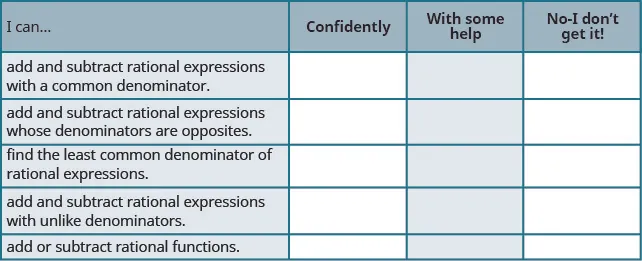After reviewing this checklist, what will you do to become confident for all objectives?

Order a print copy

As an Amazon Associate we earn from qualifying purchases.